# php数组获取值的方法有哪些php数组获取值的方法

```<?php
\$arr= array("香蕉","苹果","梨子","橙子","橘子","榴莲");
var_dump(\$arr);
//获取数组中的第一个元素
\$first = array_shift(\$arr);
echo "数组的第一个元素为：".\$first;
?>```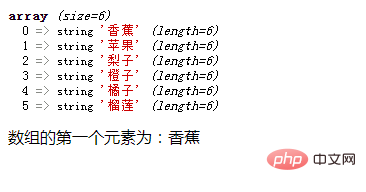```<?php
\$arr= array("香蕉","苹果","梨子","橙子","橘子","榴莲");
var_dump(\$arr);
//获取数组中的第一个元素
\$first = array_pop(\$arr);
echo "数组的最后一个元素为：".\$first;
?>```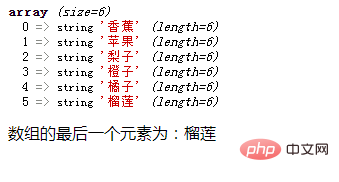```<?php
\$arr=array("a"=>"red","b"=>"green","c"=>"blue","d"=>"yellow");
\$rand = array_rand(\$arr,3);
foreach(\$rand as \$val){
\$arr2[\$val]=\$arr[\$val];
}
var_dump(\$arr2);
?>```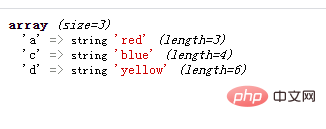```<?php
\$arr = array(10,12,20,25,24);
echo "原数组：";
var_dump(\$arr);

echo "截取的数组片段：";
\$result = array_splice(\$arr,2);  //截取从下标2开始的全部数组元素
var_dump(\$result);

\$arr = array(10,12,20,25,24);
\$result = array_splice(\$arr,1,2);//截取从下标1开始的两个元素
var_dump(\$result);

?>```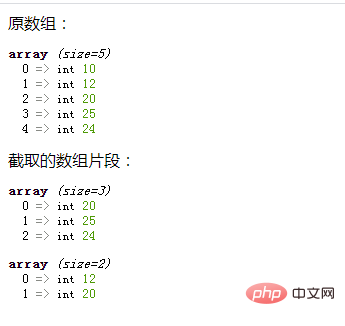```<?php
\$arr = array(10,12,20,25,24);
echo "原数组：";
var_dump(\$arr);

echo "截取的数组片段：";
\$result = array_slice(\$arr,2); //截取从下标2开始的全部数组元素
var_dump(\$result);

\$result = array_slice(\$arr,1,2); //截取从下标1开始的两个元素
var_dump(\$result);

?>```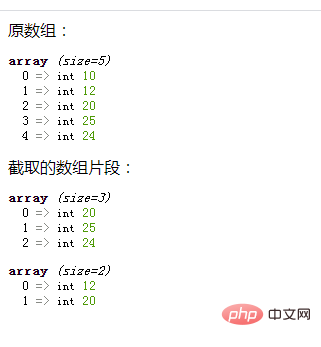• 相关标签：php 数组 获取值
• 相关文章

相关视频